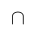Select Page

# CBSE 12 Science Solutions for MCQ Maths Probability in English

CBSE 12 Science Solutions for MCQ Maths Probability in English to enable students to get Solutions in a narrative video format for the specific question.

Expert Teacher provides CBSE 12 Science Solutions for MCQ Maths Probability through Video Solutions in English language. This video solution will be useful for students to understand how to write an answer in exam in order to score more marks. This teacher uses a narrative style for a question from Probability not only to explain the proper method of answering question, but deriving right answer too.

Please find the question below and view the Solution in a narrative video format.

Question:

Solution Video in English:

You can select video Solutions from other languages also. Please check Solutions in ( Hindi )

## Similar Questions from CBSE, 12th Science, Maths, Probability

Question 2 : A class has 15 students whose ages are 14, 17, 15, 14, 21, 17, 19, 20, 16, 18, 20, 17, 16, 19 and 20 years. One student is selected in such a manner that each has the same chance of being chosen and the age X of the selected student is recorded. What is the probability of the random variable X? Find the mean.   (View Answer Video)

Question 3 : If P(F) = 0.35 and=0.85 and E and F are independent events. Find P(E).  (View Answer Video)

Question 4 : If A and B are two events such that P(A)=0.3, P(B)=0.6 and P(B/A)=0.5, find P(AB). (View Answer Video)

Question 5 : If P(A)=0.8, P(B)=0.5 and=0.4, find. (View Answer Video)

### Integrals

Question 1 :(View Answer Video)

Question 2 : Evaluate :(View Answer Video)

Question 3 : Find :(View Answer Video)

Question 4 : Find :(View Answer Video)

Question 5 : Evaluate :(View Answer Video)

### Relations and Functions

Question 1 :  If f(x) = ax + b and g(x) = cx + d, then f[g(x)] – g[f(x)] is equivalent to, (View Answer Video)

Question 2 : Letdefined as f(x) = x be an identity function. Then, (View Answer Video)

Question 3 : Functionsare defined respectively, by, find(View Answer Video)

Question 4 : Number of binary sets on the setis, (View Answer Video)

Question 5 : Let A = {1, 2, 3, 4} and let R = {(2, 2), (3, 3), (4, 4), (1, 2)} be a relation on A. Then, R is, (View Answer Video)

### Determinant

Question 1 : If a, b, c are in AP, then determinant. (View Answer Video)

Question 2 :  If A is a square matrix of order 3 and |A| = 5 then |3A| = ? (View Answer Video)

Question 3 : Find the adjoint  of the matrix. (View Answer Video)

Question 4 :  Using the properties of determinants, evaluate. (View Answer Video)